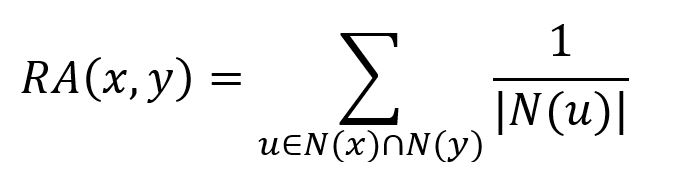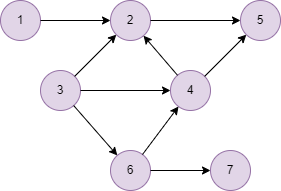# Change Nickname

Current Nickname:

Search
v4.0
v4.0

# Resource Allocation

## Overview

Resource allocation measurement assumes that every node has one unit of resources, and allocates the resources evenly to all neighbor nodes, thus the closeness of two nodes can be described by the size of resources one node allocates to the other node. This is proposed by Tao Zhou、Linyuan Lü and Yi-Cheng Zhang in 2009:

## Basic Concept

### Resource Allocation

After finding the common neighbors of two nodes, resource allocation measurement uses the sum of reciprocal of the number of neighbors of these common neighbors to determine the closeness of the two nodes, which is calculated by the following formula:where `N(x)` and `N(y)` are neighbor sets of `x` and `y` respectively, `u` is the common neighbor of `x` and `y`. The larger the value of `RA(x,y)` is, the closer the two nodes are, value of 0 indicates that the two nodes are not close.Taking the above graph as an example, the score of resource allocation of the blue and red node is the sum of the reciprocal of neighbors of the yellow and green node, which is `1/4 + 1/3 = 0.5833`.

## Special Case

### Lonely Node, Disconnected Graph

Lonely node does not have any neighbor node, the algorithm does not calculate the Resource Allocation between lonely node and any other node, either it considers the Resource Allocation of two nodes which are located in different connected components.

### Self-loop Edge

The algorithm ignores all self-loop edges when calculating neighbor nodes.

### Directed Edge

For directed edges, the algorithm ignores the direction of edges but calculates them as undirected edges.

## Results and Statistics

Take the graph below as an example, run the algorithm in the graph:Algorithm results: Calculate the Resource Allocation between node 3 and other nodes, return `node1`, `node2` and `num`

node1 node2 num
3 1 0.25
3 2 0.25
3 4 0.5833333333333333
3 5 0.5
3 6 0.25
3 7 0.3333333333333333

Algorithm statistics: N/A

## Command and Configuration

• Command: `algo(topological_link_prediction)`
• Configurations for the parameter `params()`:
Name
Type
Default
Specification Description
ids / uuids []`_id` / []`_uuid` / Mandatory IDs or UUIDs of the first set of nodes to be calculated, only need to configure one of them; every node in ids/uuids will be paired with every node in ids2/uuids2 for calculation
ids2 / uuids2 []`_id` / []`_uuid` / Mandatory IDs or UUIDs of the second set of nodes to be calculated, only need to configure one of them; every node in ids/uuids will be paired with every node in ids2/uuids2 for calculation
type string Adamic_Adar Adamic_Adar / Common_Neighbors / Preferential_Attachment / Resource_Allocation / Total_Neighbors Measurement of the closeness of the node pair; Adamic_Adar means to calculate AA index, Common_Neighbors means to calculate the number of common neighbors, Preferential_Attachment means to calculate the score of preferential attachment, Resource_Allocation means to calculate the score of resource allocation, Total_Neighbors means to calculate the number of total neighbors
limit int -1 >=-1 Number of results to return; return all results if sets to -1 or not set

## Algorithm Execution

#### 1. File Writeback

Configuration Data in Each Row
filename `node1`,`node2`,`num`

Example: Calculate the Resource Allocation of node UUID = 3 and all other nodes, write the algorithm results back to file named ra

``````algo(topological_link_prediction).params({
uuids: ,
uuids2: [1,2,4,5,6,7],
type: "Resource_Allocation"
}).write({
file:{
filename: "ra"
}
})
``````

#### 2. Property Writeback

Not supported by this algorithm.

#### 3. Statistics Writeback

This algorithm has no statistics.

### Direct Return

Alias Ordinal Type
Description
Column Name
0 []perNodePair Closeness of node pair `node1`, `node2`, `num`

Example: Calculate the Resource Allocation of node UUID = 3 and UUID = 4, define algorithm results as alias named score and return the results

``````algo(topological_link_prediction).params({
uuids: ,
uuids2: ,
type: "Resource_Allocation"
}) as score
return score
``````

### Streaming Return

Alias Ordinal Type
Description
Column Name
0 []perNodePair Closeness of node pair `node1`, `node2`, `num`

Example: Calculate the Resource Allocation of node UUID = 1 and UUID = 5,6,7, return the results in the descending closeness score

``````algo(topological_link_prediction).params({
uuids: ,
uuids2: [5,6,7],
type: "Resource_Allocation"
}).stream() as ra
return ra order by ra.num desc
``````

### Real-time Statistics

This algorithm has no statistics.